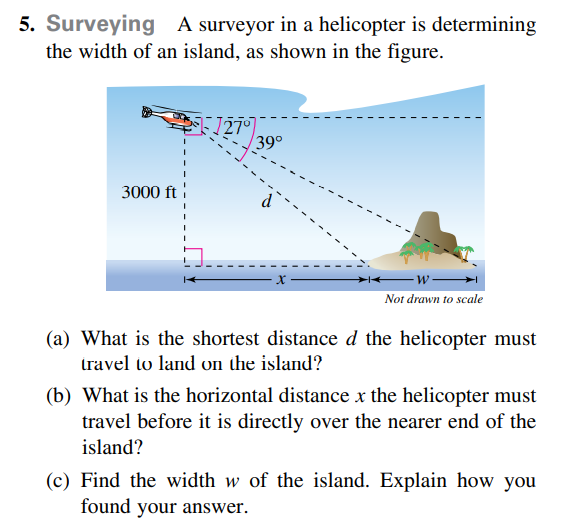### Still have math questions?

Trigonometry
Question5. Surveying A surveyor in a helicopter is determining the width of an island, as shown in the figure. (a) What is the shortest distance $$d$$ the helicopter must travel to land on the island? (b) What is the horizontal distance $$x$$ the helicopter must travel before it is directly over the nearer end of the island? (c) Find the width $$w$$ of the island. Explain how you found your answer.